##### Algebra II For Dummies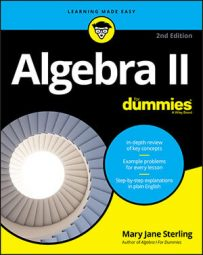The numbers zero and one have special roles in algebra — as additive and multiplicative identities, respectively. You use identities in algebra when solving equations and simplifying expressions.

You need to keep an expression equal to the same value, but you want to change its format, so you use an identity in one way or another:

• The additive identity is zero.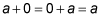Adding zero to a number doesn't change that number; it keeps its identity.

• The multiplicative identity is one.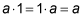Multiplying a number by one doesn't change that number; it keeps its identity.

One situation that calls for the use of the additive identity is when you want to change the format of an expression so you can factor it. For instance, take the expression x2 + 6x and add 0 to it. You get x2 + 6x + 0, which doesn't do much for you. But how about replacing that 0 with both 9 and –9? You now have x2 + 6x + 9 – 9, which you can write as (x2 + 6x + 9) – 9 and factor into (x + 3)2 – 9.

Why in the world do you want to do this? By both adding and subtracting 9, you add 0 — the additive identity. This is useful when you need to create the standard equation for a conic section such as a parabola, circle, ellipse, or hyperbola.

You use the multiplicative identity extensively when you work with fractions. Whenever you rewrite fractions with a common denominator, you actually multiply by one. If you want the fraction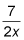to have a denominator of 6x, for example, you multiply both the numerator and denominator by 3: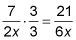Now you're ready to rock and roll with a fraction to your liking.